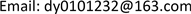1. 引言

2. 电力系统动态响应

3. 随机激励下电力系统响应的数学解析

{ x ˙ = f ( x , y )                                                                                                                                               ( 1 ) 0 = g ( x , y )                                                                                                                                                 (2)

u ˙ = − C u + δ ξ (5)

Δ y = [ − g y − 1 g x − g y − 1 g u ] [ Δ x Δ u ] (6)

[ Δ x ˙ Δ u ˙ ] = [ f x − f y g y − 1 g x − f y g y − 1 g u 0 − C ] [ Δ x Δ u ] + δ [ 0 I n u ] ξ (7)

z ˙ = A z + δ B ξ (8)

z ( t ) = ∑ i = 1 n v i u i T z ( 0 ) e σ i t sin ( ω i t + φ i ) + δ B ξ (9)

4. 实验结果与分析

The characteristic results of four generators two are

10.63221.68G1 & G2 vs. G3 & G4
21.185611.85G1 vs. G2
31.194711.18G3 vs. G3

The statistical results of active power of four generators and connected lin

G10.00447.14937.2611
G20.00516.88827.0000
G30.00686.98577.0000
G40.00736.89897.0000

The identification results of four generators two are

SSAT均值标准差SSAT均值标准差
10.63220.58080.02141.681.940.91
21.18561.17520.085211.8511.372.85
31.19471.21750.055511.1811.553.57

5. 结论

1) 随机激励下系统基本运行方式维持不变，在无新设备投运的前提下系统保持原有动态特征；

2) 采用合适的辨识技术能够从随机相应数据中提取出系统机电振荡参数，所提取参数及各机电振荡模态表征与基础运行方式吻合；

3) 实测数据分析结果表明基于随机响应数据的机电振荡特征识别对电力系统小干扰稳定在线量化评估具有重要的指导意义；